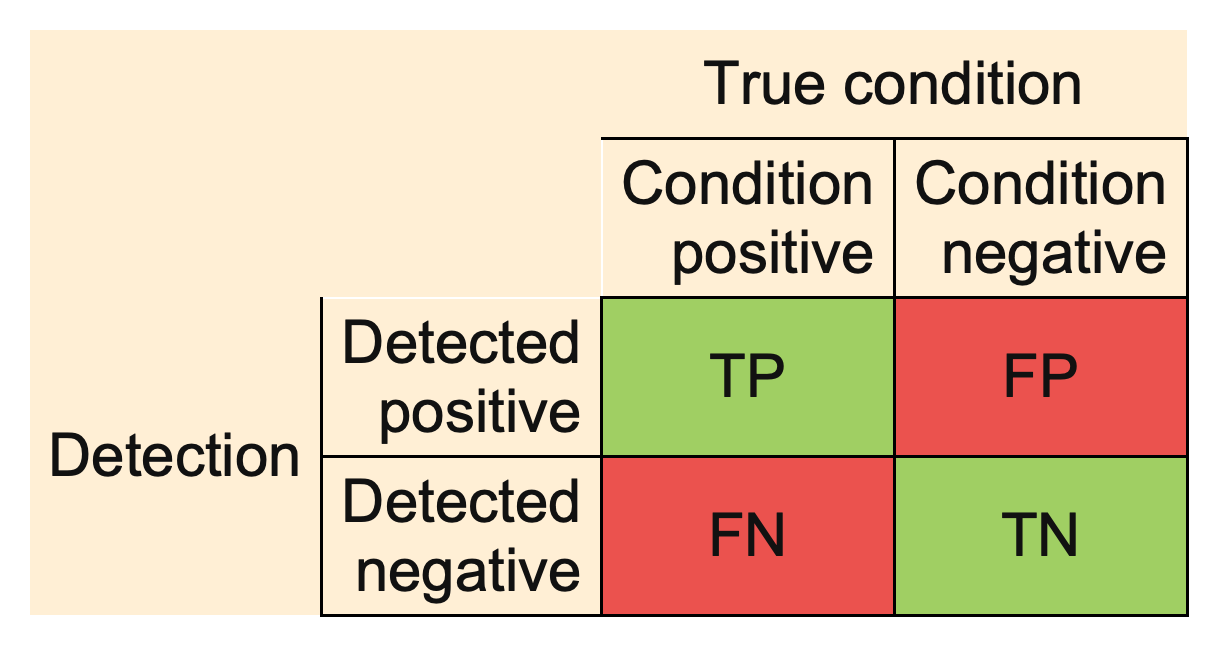ebc_allmeasures {evabic} R Documentation

## Available measures

### Description

Available measures in evabic

### Usage

ebc_allmeasures


### Format

An object of class character of length 18.

### DetailsTP

True Positive

FP

False Positive

FN

False Negative

TN

True Negative

TPR

True Positive Rate or Sensitivity or Recall or Power

TPR = \frac{TP}{TP + FN} = 1 - FNR

TNR

True Negative Rate or Specificity

TNR = \frac{TN}{FP + TN} = 1 - FPR

PPV

Positive Predictive Value or Precision

PPV = \frac{TP}{TP + FP} = 1 - FDR

NPV

Negative Predictive Value

NPV = \frac{TN}{TN + FN} = 1 - FOR

FNR

False Negative Rate or Type II Error Rate or Miss Rate

FNR = \frac{FN}{TP + FN} = 1 - TPR

FPR

False Positive Rate or Type I Errors Rate or Fall-out

FPR = \frac{FP}{FP + TN} = 1 - TNR

FDR

False Discovery Rate

FDR = \frac{FP}{FP + TP} = 1 - PPV

FOR

False Omission Rate

FOR = \frac{FN}{TN + FN} = 1 - NPV

ACC

Accuracy

ACC = \frac{TP + TN}{TP + FP + FN + TN}

BACC

Balanced Accuracy

BACC = \frac{\frac{TP}{TP + FN} + \frac{TN}{FP + TN}}{2}

F1

F1 Score

F1 = \frac{2 TP}{2TP + FP + FN} = \frac{2}{\frac{1}{TPR} + \frac{1}{PPV}}

PLR

Positive Likelihood Ratio or LR+ or Likelihood Ratio for Positive Results

PLR = \frac{TPR}{1 - TNR}

NLR

Negative Likelihood Ratio or LR- or Likelihood Ratio for Negative Results

NLR = \frac{1 - TPR}{TNR}

DOR

Diagnostic Odds Ratio

DOR = \frac{\frac{TP}{FP}}{\frac{FN}{TN}} = \frac{PLR}{NLR}

### Examples

ebc_allmeasures



[Package evabic version 0.0.3 Index]